Light Reflection and Refraction

We see a variety of objects in the world around us. However, we are unable to see anything in a dark room. On lighting up the room, things become visible. What makes things visible? During the day, the sunlight helps us to see objects. An object reflects light that falls on it. This reflected light, when received by our eyes, enables us to see things. We are able to see through a transparent medium as light is transmitted through it. There are a number of common wonderful phenomena associated with light such as image formation by mirrors, the twinkling of stars, the beautiful colours of a rainbow, bending of light by a medium and so on. A study of the properties of light helps us to explore them.

By observing the common optical phenomena around us, we may conclude that light seems to travel in straight lines. The fact that a small source of light casts a sharp shadow of an opaque object points to this straight-line path of light, usually indicated as a ray of light.

More to Know!

If an opaque object on the path of light becomes very small, light has a tendency to bend around it and not walk in a straight line – an effect known as the diffraction of light. Then the straight-line treatment of optics using rays fails. To explain phenomena such as diffraction, light is thought of as a wave, the details of which you will study in higher classes. Again, at the beginning of the 20th century, it became known that the wave theory of light often becomes inadequate for treatment of the interaction of light with matter, and light often behaves somewhat like a stream of particles. This confusion about the true nature of light continued for some years till a modern quantum theory of light emerged in which light is neither a ‘wave’ nor a ‘particle’ – the new theory reconciles the particle properties of light with the wave nature.

In this Chapter, we shall study the phenomena of reflection and refraction of light using the straight-line propagation of light. These basic concepts will help us in the study of some of the optical phenomena in nature. We shall try to understand in this Chapter the reflection of light by spherical mirrors and refraction of light and their application in real life situations.

10.1 Reflection of light

A highly polished surface, such as a mirror, reflects most of the light falling on it. You are already familiar with the laws of reflection of light.

Let us recall these laws –

1. The angle of incidence is equal to the angle of reflection, and
2. The incident ray, the normal to the mirror at the point of incidence and the reflected ray, all lie in the same plane.

These laws of reflection are applicable to all types of reflecting surfaces including spherical surfaces. You are familiar with the formation of image by a plane mirror. What are the properties of the image? Image formed by a plane mirror is always virtual and erect. The size of the image is equal to that of the object. The image formed is as far behind the mirror as the object is in front of it. Further, the image is laterally inverted. How would the images be when the reflecting surfaces are curved? Let us explore.

Activity 10.1

• Take a large shining spoon. Try to view your face in its curved surface.
• Do you get the image? Is it smaller or larger?
• Move the spoon slowly away from your face. Observe the image. How does it change?
• Reverse the spoon and repeat the Activity. How does the image look like now?
• Compare the characteristics of the image on the two surfaces.

The curved surface of a shining spoon could be considered as a curved mirror. The most commonly used type of curved mirror is the spherical mirror. The reflecting surface of such mirrors can be considered to form a part of the surface of a sphere. Such mirrors, whose reflecting surfaces are spherical, are called spherical mirrors. We shall now study about spherical mirrors in some detail.

10.2 Spherical mirrors

The reflecting surface of a spherical mirror may be curved inwards or outwards. A spherical mirror, whose reflecting surface is curved inwards, that is, faces towards the centre of the sphere, is called a concave mirror. A spherical mirror whose reflecting surface is curved outwards, is called a convex mirror. The schematic representation of these mirrors is shown in Fig. 10.1. You may note in these diagrams that the back of the mirror is shaded.

You may now understand that the surface of the spoon curved inwards can be approximated to a concave mirror and the surface of the spoon bulged outwards can be approximated to a convex mirror.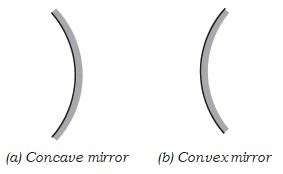Figure 10.1 Schematic representation of spherical mirrors; the shaded side is non-reflecting.

Before we move further on spherical mirrors, we need to recognise and understand the meaning of a few terms. These terms are commonly used in discussions about spherical mirrors. The centre of the reflecting surface of a spherical mirror is a point called the pole. It lies on the surface of the mirror. The pole is usually represented by the letter P.

The reflecting surface of a spherical mirror forms a part of a sphere. This sphere has a centre. This point is called the centre of curvature of the spherical mirror. It is represented by the letter C. Please note that the centre of curvature is not a part of the mirror. It lies outside its reflecting surface. The centre of curvature of a concave mirror lies in front of it. However, it lies behind the mirror in case of a convex mirror. You may note this in Fig.10.2 (a) and (b). The radius of the sphere of which the reflecting surface of a spherical mirror forms a part, is called the radius of curvature of the mirror. It is represented by the letter R. You may note that the distance PC is equal to the radius of curvature. Imagine a straight line passing through the pole and the centre of curvature of a spherical mirror. This line is called the principal axis. Remember that principal axis is normal to the mirror at its pole. Let us understand an important term related to mirrors, through an Activity.

Activity 10.2

CAUTION: Do not look at the Sun directly or even into a mirror reflecting sunlight. It may damage your eyes.

• Hold a concave mirror in your hand and direct its reflecting surface towards the Sun.
• Direct the light reflected by the mirror on to a sheet of paper held close to the mirror.
• Move the sheet of paper back and forth gradually until you find on the paper sheet a bright, sharp spot of light.
• Hold the mirror and the paper in the same position for a few minutes. What do you observe? Why?

The paper at first begins to burn producing smoke. Eventually it may even catch fire. Why does it burn? The light from the Sun is converged at a point, as a sharp, bright spot by the mirror. In fact, this spot of light is the image of the Sun on the sheet of paper. This point is the focus of the concave mirror. The heat produced due to the concentration of sunlight ignites the paper. The distance of this image from the position of the mirror gives the approximate value of focal length of the mirror. Let us try to understand this observation with the help of a ray diagram. Observe Fig.10.2 (a) closely.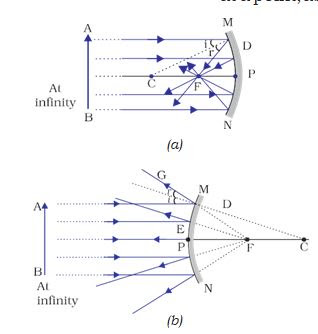Figure 10.2 (a) Concave mirror (b) Convex mirror

A number of rays parallel to the principal axis are falling on a concave mirror. Observe the reflected rays. They are all meeting/intersecting at a point on the principal axis of the mirror. This point is called the principal focus of the concave mirror. Similarly, observe Fig. 10.2 (b). How are the rays parallel to the principal axis, reflected by a convex mirror? The reflected rays appear to come from a point on the principal axis. This point is called the principal focus of the convex mirror. The principal focus is represented by the letter F. The distance between the pole and the principal focus of a spherical mirror is called the focal length. It is represented by the letter f.

The reflecting surface of a spherical mirror is by-and-large spherical. The surface, then, has a circular outline. The diameter of the reflecting surface of spherical mirror is called its aperture. In Fig.10.2, distance MN represents the aperture. We shall consider in our discussion only such spherical mirrors whose aperture is much smaller than its radius of curvature.

Is there a relationship between the radius of curvature R, and focal length f, of a spherical mirror? For spherical mirrors of small apertures, the radius of curvature is found to be equal to twice the focal length. We put this as R = 2f . This implies that the principal focus of a spherical mirror lies midway between the pole and centre of curvature.

10.2.1  Image Formation by Spherical Mirrors

You have studied about the image formation by plane mirrors. You also know the nature, position and relative size of the images formed by them. How about the images formed by spherical mirrors? How can we locate the image formed by a concave mirror for different positions of the object? Are the images real or virtual? Are they enlarged, diminished or have the same size? We shall explore this with an Activity.

Activity 10.3

You have already learnt a way of determining the focal length of a concave mirror. In Activity 10.2, you have seen that the sharp bright spot of light you got on the paper is, in fact, the image of the Sun. It was a tiny, real, inverted image. You got the approximate focal length of the concave mirror by measuring the distance of the image from the mirror.

• Take a concave mirror. Find out its approximate focal length in the way described above. Note down the value of focal length. (You can also find it out by obtaining image of a distant object on a sheet of paper.)
• Mark a line on a Table with a chalk. Place the concave mirror on a stand. Place the stand over the line such that its pole lies over the line.
• Draw with a chalk two more lines parallel to the previous line such that the distance between any two successive lines is equal to the focal length of the mirror. These lines will now correspond to the positions of the points P, F and C, respectively. Remember For a spherical mirror of small aperture, the principal focus F lies mid-way between the pole P and the centre of curvature C.
• Keep a bright object, say a burning candle, at a position far beyond

C. Place a paper screen and move it in front of the mirror till you obtain a sharp bright image of the candle flame on it.

• Observe the image carefully. Note down its nature, position and relative size with respect to the object size.
• Repeat the activity by placing the candle – (a) just beyond C,

(b) at C, (c) between F and C, (d) at F, and (e) between P and F.

• In one of the cases, you may not get the image on the screen. Identify the position of the object in such a case. Then, look for its virtual image in the mirror itself.
• Note down and tabulate your observations.

You will see in the above Activity that the nature, position and size of the image formed by a concave mirror depends on the position of the object in relation to points P, F and C. The image formed is real for some positions of the object. It is found to be a virtual image for a certain other position. The image is either magnified, reduced or has the same size, depending on the position of the object. A summary of these observations is given for your reference in Table 10.1.

Table 10.1 Image formation by a concave mirror for different positions of the object10.2.2 Representation of Images Formed by Spherical Mirrors Using Ray Diagrams

We can also study the formation of images by spherical mirrors by drawing ray diagrams. Consider an extended object, of finite size, placed in front of a spherical mirror. Each small portion of the extended object acts like a point source. An infinite number of rays originate from each of these points. To construct the ray diagrams, in order to locate the image of an object, an arbitrarily large number of rays emanating from a point could be considered. However, it is more convenient to consider only two rays, for the sake of clarity of the ray diagram. These rays are so chosen that it is easy to know their directions after reflection from the mirror.

The intersection of at least two reflected rays give the position of image of the point object. Any two of the following rays can be considered for locating the image.

(i) A ray parallel to the principal axis , after reflection, will pass through the principal focus in case of a concave mirror or appear to diverge from the principal focus in case of a convex mirror. This is illustrated in Fig.10.3 (a) and (b).(ii) A ray passing through the principal focus of a concave mirror or a ray which is directed towards the principal focus of a convex mirror, after reflection, will emerge parallel to the principal axis. This is illustrated in Fig.10.4 (a) and (b).Figure 10.4

(iii) A ray passing through the centre of curvature of a concave mirror or directed in the direction of the centre of curvature of a convex mirror, after reflection, is reflected back along the same path. This is  illustrated in Fig.10.5 (a)  and (b). The light rays come back along the same path because the incident rays fall on the mirror along the normal to the reflecting surface.

Figure 10.5(iv) A ray incident obliquely to the principal axis, towards a point P (pole of the mirror), on the concave mirror [Fig. 10.6 (a)] or a convex mirror [Fig. 10.6 (b)], is reflected obliquely. The incident and reflected rays follow the laws of reflection at the point of incidence (point P), making equal angles with the principal axis.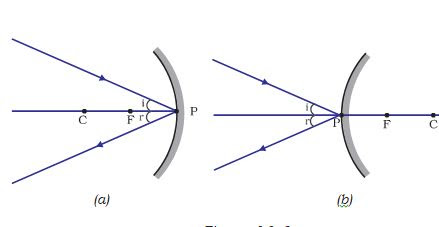Figure 10.6

Remember that in all the above cases the laws of reflection are followed. At the point of incidence, the incident ray is reflected in such a way that the angle of reflection equals the angle of incidence.

I(a) mage formation by Concave Mirror

Figure 10.7 illustrates the ray diagrams for the formation of image by a concave mirror for various positions of the object.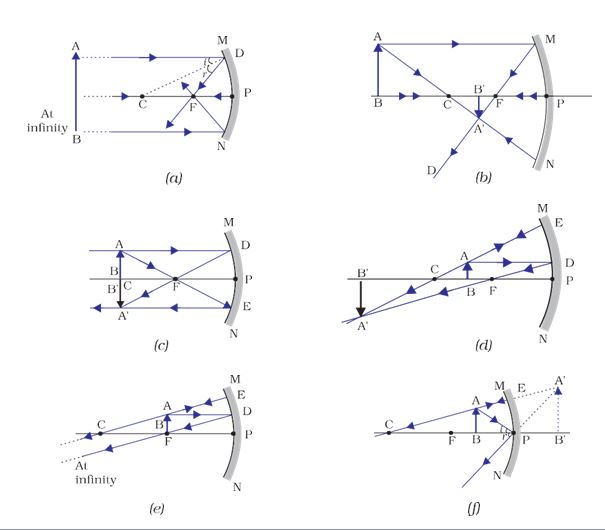Figure 10.7 Ray diagrams for the image formation by a concave mirror

Activity 10.4

• Draw neat ray diagrams for each position of the object shown in Table 10.1.
• You may take any two of the rays mentioned in the previous section for locating the image.
• Compare your diagram with those given in Fig. 10.7.
• Describe the nature, position and relative size of the image formed in each case.
• Tabulate the results in a convenient format.

Uses of concave mirrors

Concave mirrors are commonly used in torches, search-lights and vehicles headlights to get powerful parallel beams of light. They are often used as shaving mirrors to see a larger image of the face. The dentists use concave mirrors to see large images of the teeth of patients. Large concave mirrors are used to concentrate sunlight to produce heat in solar furnaces.

(b) Image formation by a Convex Mirror

We studied the image formation by a concave mirror. Now we shall study the formation of image by a convex mirror.

Activity 10.5

• Take a convex mirror. Hold it in one hand.
• Hold a pencil in the upright position in the other hand.
• Observe the image of the pencil in the mirror. Is the image erect or inverted? Is it diminished or enlarged?
• Move the pencil away from the mirror slowly. Does the image become smaller or larger?
• Repeat this Activity carefully. State whether the image will move closer to or farther away from the focus as the object is moved away from the mirror?

We consider two positions of the object for studying the image formed by a convex mirror. First is when the object is at infinity and the second position is when the object is at a finite distance from the mirror. The ray diagrams for the formation of image by a convex mirror for these two positions of the object are shown in Fig.10.8 (a) and (b), respectively. The results are summarised in Table 10.2.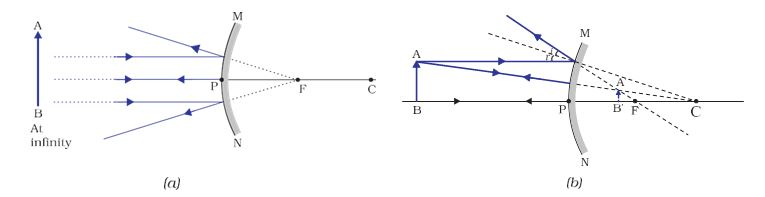Figure 10.8 Formation of image by a convex mirror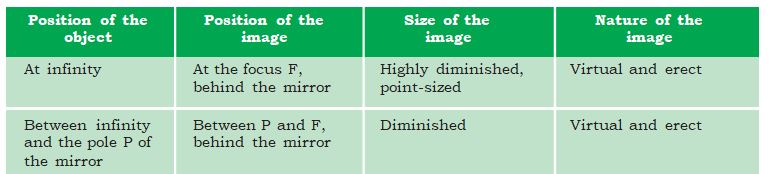Table 10.2 Nature, position and relative size of the image formed by a convex mirror

You have so far studied the image formation by a plane mirror, a concave mirror and a convex mirror. Which of these mirrors will give the full image of a large object? Let us explore through an Activity.

Activity 10.6

• Observe the image of a distant object, say a distant tree, in a plane mirror.
• Could you see a full-length image?
• Try with plane mirrors of different sizes. Did you see the entire object in the image?
• Repeat this Activity with a concave mirror. Did the mirror show full length image of the object?
• Now try using a convex mirror. Did you succeed? Explain your observations with reason.

You can see a full-length image of a tall building/tree in a small convex mirror. One such mirror is fitted in a wall of Agra Fort facing Taj Mahal. If you visit the Agra Fort, try to observe the full image of Taj Mahal. To view distinctly, you should stand suitably at the terrace adjoining the wall.

Uses of convex mirrors

Convex mirrors are commonly used as rear -view (wing) mirrors in vehicles. These mirrors are fitted on the sides of the vehicle, enabling the driver to see traffic behind him/her to facilitate safe driving. Convex mirrors are preferred because they always give an erect, though diminished, image. Also, they have a wider field of view as they are curved outwards. Thus, convex mirrors enable the driver to view much larger area than would be possible with a plane mirror.

Questions

1. Define the principal focus of a concave mirror.
Ans:-
The principle focus of concave mirror is a point on its principle axis to which all the light rays which are parallel and close to the axis, converge after reflection from the mirror.

2. The radius of curvature of a spherical mirror is 20 cm. What is its focal length?
Ans:-
Radius of curvature (®) = 20 cm
Radius of curvature of the spherical mirror = 2 × Focal length (f)R = 2f
f= R/2 = 20 / 2 = 10
The focal length of the spherical mirror is 10 cm.

3. Name a mirror that can give an erect and enlarged image of an object.
Ans:-
A concave mirror gives an erect and enlarged image of an object held between pole and principal focus of the mirror.

4. Why do we prefer a convex mirror as a rear-view mirror in vehicles?
Ans:-
Convex mirror is preferred as a rear-view mirror in cars and vehicles as it gives a wider field of view, which helps the driver to see most of the traffic behind him. Convex mirrors always form an erect, virtual, and diminished image of the objects placed in front of it.